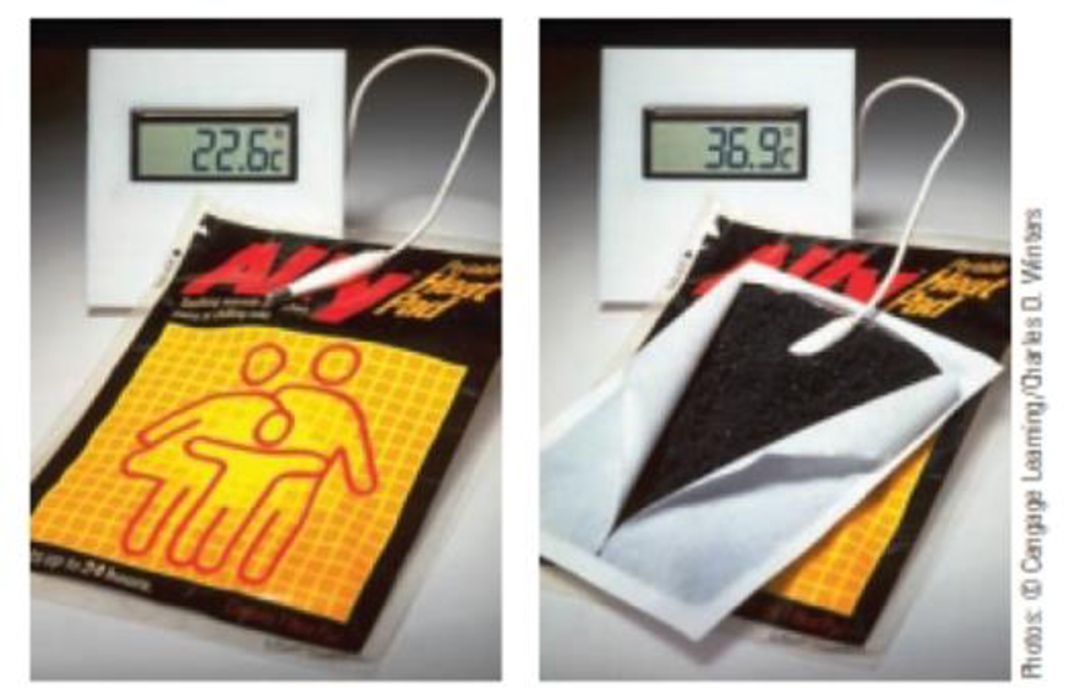# On a cold day, you can warm your hands with a “heat pad,” a device that uses the oxidation of iron to produce energy as heat. 4 Fe(s) + 3 O 2 (g) → 2 Fe 2 O 3 (s) A hand warmer uses the oxidation of iron as a source of thermal energy. What mass of iron is needed to supply the energy required to warm 15 mL of water ( d = 1.00 g/mL) from 23 °C to 37 °C?### Chemistry & Chemical Reactivity

9th Edition
John C. Kotz + 3 others
Publisher: Cengage Learning
ISBN: 9781133949640

#### Solutions

Chapter
Section### Chemistry & Chemical Reactivity

9th Edition
John C. Kotz + 3 others
Publisher: Cengage Learning
ISBN: 9781133949640
Chapter 5, Problem 100IL
Textbook Problem
54 views

## On a cold day, you can warm your hands with a “heat pad,” a device that uses the oxidation of iron to produce energy as heat.4 Fe(s) + 3 O2(g) → 2 Fe2O3(s)A hand warmer uses the oxidation of iron as a source of thermal energy.What mass of iron is needed to supply the energy required to warm 15 mL of water (d = 1.00 g/mL) from 23 °C to 37 °C?

Interpretation Introduction

Interpretation:

The required amount of Fe to warm 15mL of water has to be calculated.

Concept Introduction:

Standard enthalpy of the reaction,ΔrHo, is the change in enthalpy that happens when matter is transformed by a given chemical reaction, when all reactants and products are in their standard states. Enthalpy of the reaction,ΔrH, is the change in enthalpy that happens when matter is transformed by a given chemical reaction

Heat energy required to raise the temperature of 1g of substance by 1K..Energy gained or lost can be calculated using the below equation.

q=C×m×ΔT

Where, q= energy gained or lost for a given mass of substance (m), C =specific heat capacity,ΔT= change in temperature.

The standard enthalpy change of combustion of a compound is the enthalpy change which occurs when one gram of the compound is burned completely in oxygen under standard conditions, and with everything in its standard state.

ΔrH0=ΣnΔfH0(products)-ΣnΔfH0(reactants)

### Explanation of Solution

Given,

ΔfH Of Fe=0

ΔfH Of oxygen = 0

ΔfH Of Fe2O3=-822.2kJ/mol

ΔrH0=ΣnΔfH0(products)-ΣnΔfH0(reactants)

ΔrH= (2mol×-822.2kJ/mol)- (0)

ΔrH=-1644kJ

Heat required to warm 15mL water

Specific capacity of heat of iron is 0

### Still sussing out bartleby?

Check out a sample textbook solution.

See a sample solution

#### The Solution to Your Study Problems

Bartleby provides explanations to thousands of textbook problems written by our experts, many with advanced degrees!

Get Started

Find more solutions based on key concepts
A built environment can support physical activity with a. safe biking and walking lanes. b. public parks. c. fr...

Nutrition: Concepts and Controversies - Standalone book (MindTap Course List)

Review. An object with a mass of m = 5.10 kg is attached to the free end of a light string wrapped around a ree...

Physics for Scientists and Engineers, Technology Update (No access codes included)

What is the pH of a 0.10M solution of acetic acid (Ka=1.8105)?

Introductory Chemistry: An Active Learning Approach

How do heavy metals enter the food chain? What can be the results?

Oceanography: An Invitation To Marine Science, Loose-leaf Versin